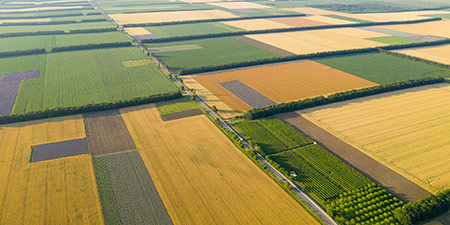# Convert Area Units | Hectare Meter Ar Mile

The area converter helps you to convert all common area units into the other unit of area you are looking for. Behind the help buttons, you will find a lot of interesting information about the selected area measure.

## Area CalculatorHow big is a hectare? How many square meters are in a square kilometer? How big is an ar? You can clarify such and many similar questions with the help of the area converter. In addition to the conversion of all common metrics, i.e., meter or square meter based area measures, the area calculator also supports the conversion into many area measures from the Anglo-American area, such as square miles, acres or square yards.

By the way, you can also learn about Calculate area of a triangle, the Calculate area of a circle or Calculate square meters of rectangular areas.

## Operating Aid

Enter the unit of area to be converted and select from and to which unit of area this value is to be converted. The area converter provides the common metric and Anglo-American area measures for the calculation for this purpose. Further information on the different area units is hidden behind the help buttons of the area converter marked with a question mark.

## The Different Area Units

Below you will find information about all area units that can be converted in the area converter.

### Square Kilometer

One square kilometer (km²; spelled kilometre in Commonwealth English and kilometer in American English) corresponds to the area of a square with a side length of one kilometer.

1 km² = 1000 m × 1000 m

### Hectar

One hectare (ha) is equal to the area of a square with sides 100 meters long.

1 ha = 100 m × 100 m

### Ar

One Ar (a) (Are in Switzerland) corresponds to the area of a square with a side length of 10 meters.

1 a = 10 m × 10 m

### Square Meter

One square meter (m²; spelled metre in Commonwealth English and meter in American English) is equal to the area of a square with a side length of one meter.

1 m² = 1 m × 1 m

### Square Decimeter

One square decimeter (dm²; spelled decimetre in Commonwealth English and decimeter in American English) is equal to the area of a square with a side length of 10 cm.

1 dm² = 10 cm × 10 cm

### Square Centimeter

One square centimeter (cm²; spelled centimetre in Commonwealth English and centimeter in American English) is equal to the area of a square with a side length of 1 cm.

1 cm² = 1 cm × 1 cm

### Square Millimeter

One square millimeter (mm²; spelled millimetre in Commonwealth English and millimeter in American English) corresponds to the area of a square with a side length of 1 mm.

1 mm² = 1 mm × 1 mm

### Square Mile

A square mile (mi²) or sq mi, is equal to the area of a square with a side length of one mile (1,609.34 meters).

### Quarter

A quarter section is equal to one quarter of a square mile, i.e., the area of a square with a side length of half a mile (804.67 meters).

### Acre

An acre (ac) is equal to one 160th part of a quarter section or one 640th part of a square mile. Thus, the acre has the area of a square with a side length of about 63.62 meters.

### Square Yard

A square yard (yd²) is equal to the area of a square with a side length of one yard (0.9144 meters).

### Square Foot

A square foot (ft²; plural square feet) is equal to the area of a square with a side length of one foot (30.48 cm).

### Square Inch

One square inch (in²) is equal to the area of a square with a side length of one inch (2.54 cm).

### Other area units

There are other SI units of area that use a fraction of the square meter. For this purpose, so-called unit prefixes, i.e., prefixes for the square meter, are formed, as shown in some of the previous examples.

The following table gives an overview of the prefix usage for the fractions of a square meter:

.
Symbol Name Value
Square meter 100
dm² Square decimeter 10-1
cm² Square centimeter 10-2
mm² Square millimetre 10-3
µm² Square micrometer 10-6
nm² Square nanometer 10-9
pm² Square pikometer 10-12
fm² Square femtometer 10-15
am² Square actometer 10-18
zm² Square ceptometer 10-21
ym² Square yoctometer 10-24

## Source information

As source for the information in the 'Area Units' category, we have used in particular:

## Last update on February 20, 2023

The pages of the 'Area Units' category were last editorially reviewed by Michael Mühl on February 20, 2023. They all correspond to the current status.

### Previous changes on November 28, 2022

• 14.11.2022: Publication of Area Converter together with associated texts.
• Editorial revision of all texts in this category